# Solid harmonics

In mathematics, solid harmonics are defined as solutions of the Laplace equation in spherical polar coordinates. There are two kinds of solid harmonic functions: the regular solid harmonics$R^m_\ell(\mathbf{r})$, which vanish at the origin, and the irregular solid harmonics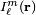$I^m_{\ell}(\mathbf{r})$, which have an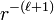$r^{-(\ell+1)}$ singularity at the origin. Both sets of functions play an important role in potential theory. Regular solid harmonics appear in chemistry in the form of s, p, d, etc. atomic orbitals and in physics as multipoles. Irregular harmonics appear in the expansion of scalar fields in terms of multipoles.

Both kinds of solid harmonics are simply related to spherical harmonics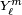$Y^m_\ell$ (normalized to unity),$R^m_{\ell}(\mathbf{r}) \equiv \sqrt{\frac{4\pi}{2\ell+1}}\; r^\ell Y^m_{\ell}(\theta,\varphi), \qquad I^m_{\ell}(\mathbf{r}) \equiv \sqrt{\frac{4\pi}{2\ell+1}} \; \frac{ Y^m_{\ell}(\theta,\varphi)}{r^{\ell+1}} .$

##  Derivation, relation to spherical harmonics

The following vector operator plays a central role in this section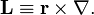$\mathbf{L} \equiv \mathbf{r} \times \mathbf{\nabla}.$

Parenthetically, we remark that in quantum mechanics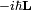$-i\hbar \mathbf{L}$ is the orbital angular momentum operator, where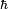$\hbar\,$ is Planck's constant divided by 2π. In quantum mechanics the momentum operator is proportional to the gradient,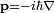$\mathbf{p}= -i\hbar\mathbf{\nabla}$, so that L is proportional to r×p, the orbital angular momentum operator.

By using the relations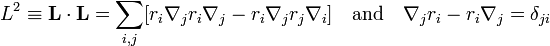$L^2 \equiv \mathbf{L}\cdot\mathbf{L} = \sum_{i,j} [r_i\nabla_j r_i \nabla_j - r_i\nabla_j r_j \nabla_i]\quad \hbox{and}\quad \nabla_j r_i - r_i\nabla_j = \delta_{ji}$

one can derive that$L^2 = r^2 \nabla^2 - (\mathbf{r}\cdot\mathbf{\nabla} )^2 - \mathbf{r}\cdot\mathbf{\nabla}.$

Expression in spherical polar coordinates gives:$\mathbf{r}\cdot \mathbf{\nabla} = r\frac{\partial}{\partial r}$

and$(\mathbf{r}\cdot\mathbf{\nabla} )^2 + \mathbf{r}\cdot\mathbf{\nabla} = \frac{1}{r} \frac{\partial^2}{\partial r^2} r .$

It can be shown by expression of L in spherical polar coordinates that L² does not contain a derivative with respect to r. Hence upon division of L² by r² the position of 1/r² in the resulting expression is irrelevant. After these preliminaries we find that the Laplace equation ∇² Φ = 0 can be written as$\nabla^2\Phi(\mathbf{r}) = \left(\frac{1}{r} \frac{\partial^2}{\partial r^2}r + \frac{L^2}{r^2} \right)\Phi(\mathbf{r}) = 0 , \qquad \mathbf{r} \ne \mathbf{0}.$

It is known that spherical harmonics Yml are eigenfunctions of L²: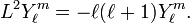$L^2 Y^m_{\ell} = -\ell(\ell+1) Y^m_{\ell}.$

Substitution of Φ(r) = F(r) Yml into the Laplace equation gives, after dividing out the spherical harmonic function, the following radial equation and its general solution,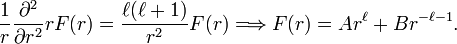$\frac{1}{r}\frac{\partial^2}{\partial r^2}r F(r) =\frac{\ell(\ell+1)}{r^2} F(r) \Longrightarrow F(r) = A r^\ell + B r^{-\ell-1}.$

The particular solutions of the total Laplace equation are regular solid harmonics: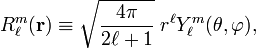$R^m_{\ell}(\mathbf{r}) \equiv \sqrt{\frac{4\pi}{2\ell+1}}\; r^\ell Y^m_{\ell}(\theta,\varphi),$

and irregular solid harmonics:$I^m_{\ell}(\mathbf{r}) \equiv \sqrt{\frac{4\pi}{2\ell+1}} \; \frac{ Y^m_{\ell}(\theta,\varphi)}{r^{\ell+1}} .$

Racah's normalization (also known as Schmidt's semi-normalization) is applied to both functions$\int_{0}^{\pi}\sin\theta\, d\theta \int_0^{2\pi} d\varphi\; R^m_{\ell}(\mathbf{r})^*\; R^m_{\ell}(\mathbf{r}) = \frac{4\pi}{2\ell+1} r^{2\ell}$

(and analogously for the irregular solid harmonic) instead of normalization to unity. This is convenient because in many applications the Racah normalization factor appears unchanged throughout the derivations.

## Connection between regular and irregular solid harmonics

From the definitions follows immediately that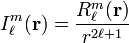$I^m_\ell(\mathbf{r})= \frac{R^m_{\ell}(\mathbf{r})}{r^{2\ell+1}}$

A more interesting relationship follows from the observation that the regular solid harmonics are homogeneous polynomials in the components x, y, and z of r. We can replace these components by the corresponding components of the gradient operator ∇. Thus, the left hand side in the following equation is well-defined:$R^m_\ell(\mathbf{\nabla})\; \frac{1}{r} = (-1)^\ell \frac{(2\ell)!}{2^\ell \ell!}\;I^m_\ell(\mathbf{r}), \qquad r\ne 0.$

For a proof see Biedenharn and Louck (1981), p. 312.

The translation of the regular solid harmonic gives a finite expansion,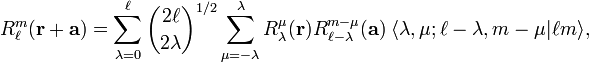$R^m_\ell(\mathbf{r}+\mathbf{a}) = \sum_{\lambda=0}^\ell\binom{2\ell}{2\lambda}^{1/2} \sum_{\mu=-\lambda}^\lambda R^\mu_{\lambda}(\mathbf{r}) R^{m-\mu}_{\ell-\lambda}(\mathbf{a})\; \langle \lambda, \mu; \ell-\lambda, m-\mu| \ell m \rangle,$

where the Clebsch-Gordan coefficient is given by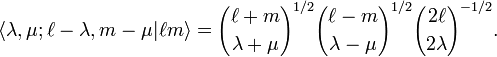$\langle \lambda, \mu; \ell-\lambda, m-\mu| \ell m \rangle = {\ell+m \choose \lambda+\mu}^{1/2} {\ell-m \choose \lambda-\mu}^{1/2} {2\ell \choose 2\lambda}^{-1/2}.$

The similar expansion for irregular solid harmonics gives an infinite series,$I^m_\ell(\mathbf{r}+\mathbf{a}) = \sum_{\lambda=0}^\infty\binom{2\ell+2\lambda+1}{2\lambda}^{1/2} \sum_{\mu=-\lambda}^\lambda R^\mu_{\lambda}(\mathbf{r}) I^{m-\mu}_{\ell+\lambda}(\mathbf{a})\; \langle \lambda, \mu; \ell+\lambda, m-\mu| \ell m \rangle$

with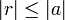$|r| \le |a|\,$. The quantity between pointed brackets is again a Clebsch-Gordan coefficient,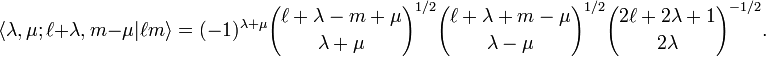$\langle \lambda, \mu; \ell+\lambda, m-\mu| \ell m \rangle = (-1)^{\lambda+\mu}{\ell+\lambda-m+\mu \choose \lambda+\mu}^{1/2} {\ell+\lambda+m-\mu \choose \lambda-\mu}^{1/2}{2\ell+2\lambda+1\choose 2\lambda}^{-1/2}.$

## Real form

By a simple linear combination of solid harmonics of ±m these functions are transformed into real functions. The real regular solid harmonics, expressed in Cartesian coordinates, are homogeneous polynomials of order l in x, y, z. The explicit form of these polynomials is of some importance. They appear, for example, in the form of spherical atomic orbitals and real multipole moments. The explicit Cartesian expression of the real regular harmonics will now be derived.

#### Linear combination

We write in agreement with the earlier definition$R_\ell^m(r,\theta,\varphi) = (-1)^{(m+|m|)/2}\; r^\ell \;\Theta_{\ell}^{|m|} (\cos\theta) e^{im\varphi}, \qquad -\ell \le m \le \ell,$

with$\Theta_{\ell}^m (\cos\theta) \equiv \left[\frac{(\ell-m)!}{(\ell+m)!}\right]^{1/2} \,\sin^m\theta\, \frac{d^m P_\ell(\cos\theta)}{d\cos^m\theta}, \qquad m\ge 0,$

where$P_\ell(\cos\theta)$ is a Legendre polynomial of order l. The m dependent phase is known as the Condon-Shortley phase.

The following expression defines the real regular solid harmonics:$\begin{pmatrix} C_\ell^{m} \\ S_\ell^{m} \end{pmatrix} \equiv \sqrt{2} \; r^\ell \; \Theta^{m}_\ell \begin{pmatrix} \cos m\varphi\\ \sin m\varphi \end{pmatrix} = \frac{1}{\sqrt{2}} \begin{pmatrix} (-1)^m & \quad 1 \\ -(-1)^m i & \quad i \end{pmatrix} \begin{pmatrix} R_\ell^{m} \\ R_\ell^{-m} \end{pmatrix}, \qquad m > 0.$

and for m = 0:$C_\ell^{0} \equiv R_\ell^{0} .$

Since the transformation is by a unitary matrix the normalization of the real and the complex solid harmonics is the same.

####  z-dependent part

Upon writing u = cos θ the mth derivative of the Legendre polynomial can be written as the following expansion in u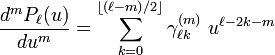$\frac{d^m P_\ell(u)}{du^m} = \sum_{k=0}^{\left \lfloor (\ell-m)/2\right \rfloor} \gamma^{(m)}_{\ell k}\; u^{\ell-2k-m}$

with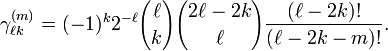$\gamma^{(m)}_{\ell k} = (-1)^k 2^{-\ell} {\ell\choose k}{2\ell-2k \choose \ell} \frac{(\ell-2k)!}{(\ell-2k-m)!}.$

Since z = r cosθ it follows that this derivative, times an appropriate power of r, is a simple polynomial in z,$\Pi^m_\ell(z)\equiv r^{\ell-m} \frac{d^m P_\ell(u)}{du^m} = \sum_{k=0}^{\left \lfloor (\ell-m)/2\right \rfloor} \gamma^{(m)}_{\ell k}\; r^{2k}\; z^{\ell-2k-m}.$

####  (x,y)-dependent part

Consider next, recalling that x = r sinθcosφ and y = r sinθsinφ,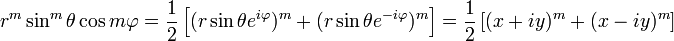$r^m \sin^m\theta \cos m\varphi = \frac{1}{2} \left[ (r \sin\theta e^{i\varphi})^m + (r \sin\theta e^{-i\varphi})^m \right] = \frac{1}{2} \left[ (x+iy)^m + (x-iy)^m \right]$

Likewise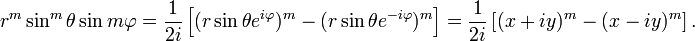$r^m \sin^m\theta \sin m\varphi = \frac{1}{2i} \left[ (r \sin\theta e^{i\varphi})^m - (r \sin\theta e^{-i\varphi})^m \right] = \frac{1}{2i} \left[ (x+iy)^m - (x-iy)^m \right].$

Further$A_m(x,y) \equiv \frac{1}{2} \left[ (x+iy)^m + (x-iy)^m \right]= \sum_{p=0}^m {m\choose p} x^p y^{m-p} \cos\left( (m-p) \frac{\pi}{2} \right)$

and$B_m(x,y) \equiv \frac{1}{2i} \left[ (x+iy)^m - (x-iy)^m \right]= \sum_{p=0}^m {m\choose p} x^p y^{m-p} \sin\left( (m-p) \frac{\pi}{2}\right).$

#### In total$C^m_\ell(x,y,z) = \left[\frac{(2-\delta_{m0}) (\ell-m)!}{(\ell+m)!}\right]^{1/2} \Pi^m_{\ell}(z)\;A_m(x,y),\qquad m=0,1, \ldots,\ell$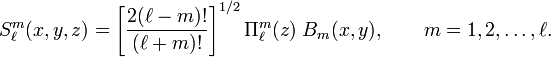$S^m_\ell(x,y,z) = \left[\frac{2 (\ell-m)!}{(\ell+m)!}\right]^{1/2} \Pi^m_{\ell}(z)\;B_m(x,y) ,\qquad m=1,2,\ldots,\ell.$

####  List of lowest functions

We list explicitly the lowest functions up to and including l = 5 . Here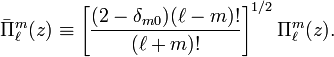$\bar{\Pi}^m_\ell(z) \equiv \left[\frac{(2-\delta_{m0}) (\ell-m)!}{(\ell+m)!}\right]^{1/2} \Pi^m_{\ell}(z) .$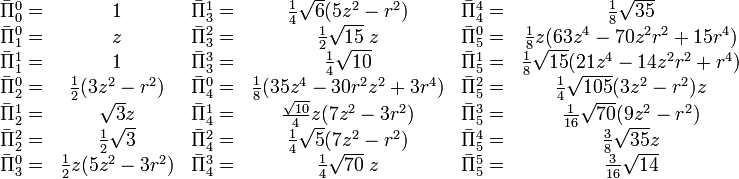$\begin{matrix} \bar{\Pi}^0_0 = & 1 & \bar{\Pi}^1_3 = & \frac{1}{4}\sqrt{6}(5z^2-r^2) & \bar{\Pi}^4_4 = & \frac{1}{8}\sqrt{35} \\ \bar{\Pi}^0_1 = & z & \bar{\Pi}^2_3 = & \frac{1}{2}\sqrt{15}\; z & \bar{\Pi}^0_5 = & \frac{1}{8}z(63z^4-70z^2r^2+15r^4) \\ \bar{\Pi}^1_1 = & 1 & \bar{\Pi}^3_3 = & \frac{1}{4}\sqrt{10} & \bar{\Pi}^1_5 = & \frac{1}{8}\sqrt{15} (21z^4-14z^2r^2+r^4) \\ \bar{\Pi}^0_2 = & \frac{1}{2}(3z^2-r^2) & \bar{\Pi}^0_4 = & \frac{1}{8}(35 z^4-30 r^2 z^2 +3r^4 ) & \bar{\Pi}^2_5 = & \frac{1}{4}\sqrt{105}(3z^2-r^2)z \\ \bar{\Pi}^1_2 = & \sqrt{3}z & \bar{\Pi}^1_4 = & \frac{\sqrt{10}}{4} z(7z^2-3r^2) & \bar{\Pi}^3_5 = & \frac{1}{16}\sqrt{70} (9z^2-r^2) \\ \bar{\Pi}^2_2 = & \frac{1}{2}\sqrt{3} & \bar{\Pi}^2_4 = & \frac{1}{4}\sqrt{5}(7z^2-r^2) & \bar{\Pi}^4_5 = & \frac{3}{8}\sqrt{35} z \\ \bar{\Pi}^0_3 = & \frac{1}{2} z(5z^2-3r^2) & \bar{\Pi}^3_4 = & \frac{1}{4}\sqrt{70}\;z & \bar{\Pi}^5_5 = & \frac{3}{16}\sqrt{14} \\ \end{matrix}$

The lowest functions$A_m(x,y)\,$ and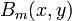$B_m(x,y)\,$ are:

m Am Bm
0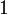$1\,$$0\,$
1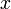$x\,$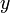$y\,$
2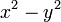$x^2-y^2\,$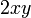$2xy\,$
3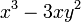$x^3-3xy^2\,$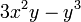$3x^2y -y^3\,$
4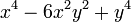$x^4 - 6x^2 y^2 +y^4\,$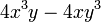$4x^3y-4xy^3\,$
5$x^5-10x^3y^2+ 5xy^4\,$$5x^4y -10x^2y^3+y^5\,$

#### Examples

Thus, for example, the angular part of one of the nine normalized spherical g atomic orbitals is: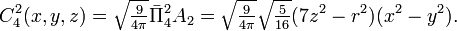$C^2_4(x,y,z) = \sqrt{{\textstyle\frac{9}{4\pi}}}\bar{\Pi}^2_4 A_2= \sqrt{{\textstyle\frac{9}{4\pi}}} \sqrt{\textstyle{\frac{5}{16}}} (7z^2-r^2)(x^2-y^2).$

One of the 7 components of a real multipole of order 3 (octupole) of a system of N charges q i is$S^1_3(x,y,z) = \bar{\Pi}^1_3 B_1= \frac{1}{4}\sqrt{6}\sum_{i=1}^N q_i (5z_i^2-r_i^2) y_i .$

## Spherical harmonics in Cartesian form

The following expresses normalized spherical harmonics in Cartesian coordinates (Condon-Shortley phase):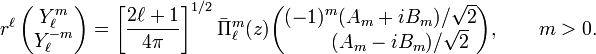$r^\ell\, \begin{pmatrix} Y_\ell^{m} \\ Y_\ell^{-m} \end{pmatrix} = \left[\frac{2\ell+1}{4\pi}\right]^{1/2} \bar{\Pi}^m_\ell(z) \begin{pmatrix} (-1)^m (A_m + i B_m)/\sqrt{2} \\ \qquad (A_m - i B_m)/\sqrt{2} \\ \end{pmatrix} , \qquad m > 0.$

and for m = 0: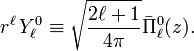$r^\ell\,Y_\ell^{0} \equiv \sqrt{\frac{2\ell+1}{4\pi}} \bar{\Pi}^0_\ell(z) .$

Here$A_m(x,y) = \sum_{p=0}^m {m \choose p} x^p y^{m-p} \cos\left( (m-p) \frac{\pi}{2}\right),$$B_m(x,y) = \sum_{p=0}^m {m\choose p} x^p y^{m-p} \sin\left( (m-p) \frac{\pi}{2} \right),$

and for m > 0: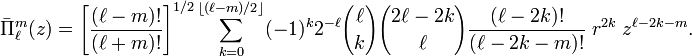$\bar{\Pi}^m_\ell(z) = \left[\frac{(\ell-m)!}{(\ell+m)!}\right]^{1/2} \sum_{k=0}^{\left \lfloor (\ell-m)/2\right \rfloor} (-1)^k 2^{-\ell} {\ell\choose k}{2\ell-2k \choose \ell} \frac{(\ell-2k)!}{(\ell-2k-m)!} \; r^{2k}\; z^{\ell-2k-m}.$

For m = 0:$\bar{\Pi}^0_\ell(z) = \sum_{k=0}^{\left \lfloor \ell/2\right \rfloor} (-1)^k 2^{-\ell} {\ell \choose k}{2\ell-2k \choose \ell} \; r^{2k}\; z^{\ell-2k}.$
##### Examples

Using the expressions for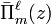$\bar{\Pi}^\ell_m(z)$,$A_m(x,y)\,$, and$B_m(x,y)\,$ listed explicitly above we obtain:$Y^1_3 = - \frac{1}{r^3} \left[{\textstyle \frac{7}{4\pi}\cdot \frac{3}{16} }\right]^{1/2} (5z^2-r^2)(x+iy) = - \left[{\textstyle \frac{7}{4\pi}\cdot \frac{3}{16}}\right]^{1/2} (5\cos^2\theta-1) (\sin\theta e^{i\varphi})$$Y^{-2}_4 = \frac{1}{r^4} \left[{\textstyle \frac{9}{4\pi}\cdot\frac{5}{32}}\right]^{1/2}(7z^2-r^2) (x-iy)^2 = \left[{\textstyle \frac{9}{4\pi}\cdot\frac{5}{32}}\right]^{1/2}(7 \cos^2\theta -1) (\sin^2\theta e^{-2 i \varphi})$